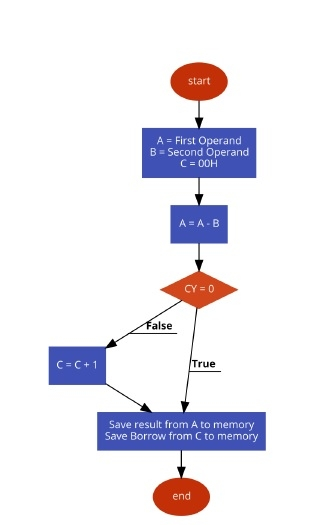# Program to Subtract two 8 Bit numbers in 8085 Microprocessor

Here we will see one 8085 program. In this program we will see how to subtract two 8-bit numbers.

Problem Statement

Write an 8085 Assembly language program to subtract two 8-bit numbers and store the result at locations 8050H and 8051H.

Discussion

In 8085, the SUB instruction is used 2’s complemented method for subtraction. When the first operand is larger, the result will be positive. It will not enable the carry flag after completing the subtraction. When the result is negative, then the result will be in 2’s complemented form and carry flag will be enabled.

We are using two numbers at location 8000H and 8001H. When the numbers are 78H and 5DH, then the result will be (78 – 5D = 1B) and when the numbers are 23H and CFH, then the result will be (23 – CF = 154) Here 1 indicates the number is negative. The actual result is 54H. It is in 2’s complemented form.

first input

Data

8000
78
8001
5D

second input

Data

8000
23
8001
CF

## Flow Diagram## Program

HEX Codes
Labels
Mnemonics
F000
0E, 00

MVI C,00H
Clear C register
F002
21, 00, 80

LXI H,8000H
F005
7E

MOV A,M
F006
23

INX H
Point to next location
F007
46

MOV B,M
F008
90

SUB B
Subtract B from A
F009
D2, 0D, F0

JNC STORE
When CY = 0, go to STORE
F00C
0C

INR C
Increase C by 1
F00D
21, 50, 80
STORE
LXI H,8050H
F010
77

MOV M,A
Store the result
F011
23

INX H
Point to next location
F012
71

MOV M,C
Store the borrow
F013
76

HLT
Terminate the program

first output

Data

8050
1B
8051
00

second output